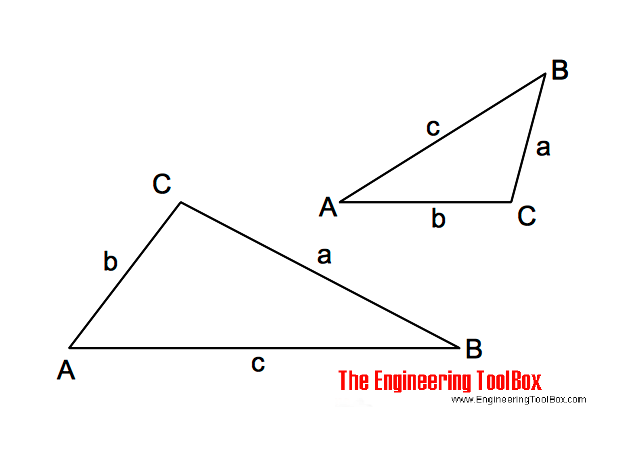Engineering ToolBox - Resources, Tools and Basic Information for Engineering and Design of Technical Applications!

# Oblique Triangle

## Calculate oblique triangles.

An oblique triangle is any triangle that is not a right angled triangle.### Triangle Three Known Values - Length and Angle Calculator

Calculate the unknown lengths and angles in a triangle. Add three known values - leave the rest of the inputs blank.

Note! - the calculator is based on the same value combinations used in the equations below. Other value combinations will not work - most triangles with three known values can be adapted to these equations. The calculator is quite simple. With strange results - check your input values.

Angle A (degrees)

Angle B (degrees)

Angle C (degrees)

Length a (m, mm, ft, in ..)

Length b (m, mm, ft, in ..)

Length c (m, mm, ft, in ..)

Area (m2, mm2, ft2, in2 .....):

(enable pop-up)

### A, B and a is known, calculate

b = a sin(B) / sin(A)                       (1a)

C = 180o - (A + B)                        (1b)

c = a sin(C) / sin(A)                       (1c)

### A, a and b is known, calculate

sin(B) = b sin(A) / a                      (2a)

C = 180o - (A + B)                      (2b)

c = a sin(C) / sin(A)                      (2c)

### a, b and C is known, calculate

tan(A) = a sin(C) / (b - a cos(C))                 (3a)

B= 180o - (A + C)                        (3b)

c = a sin(C) / sin(A)                       (3c)

### a, b and c is known, calculate

cos(A) = (b2 + c2 - a2) / (2 b c)                      (4a)

cos(B)= (a2 + c2 - b2) / (2 a c)                      (4b)

C = 180o - (A + B)                              (4c)

### a, b, c, A, B and C is known, calculate area

s = (a + b + c) / 2                        (5a)

area = (s (s - a) (s- b) (s - c))1/2                     (5b)

area = b c sin(A) / 2                           (5c)

area = a2 sin(B) sin(C) / (2 sin(A))                     (5d)

## Related Topics

• Mathematics - Mathematical rules and laws - numbers, areas, volumes, exponents, trigonometric functions and more.

## Engineering ToolBox - SketchUp Extension - Online 3D modeling!

Add standard and customized parametric components - like flange beams, lumbers, piping, stairs and more - to your Sketchup model with the Engineering ToolBox - SketchUp Extension - enabled for use with the amazing, fun and free SketchUp Make and SketchUp Pro .Add the Engineering ToolBox extension to your SketchUp from the SketchUp Pro Sketchup Extension Warehouse!

Translate

## Privacy

We don't collect information from our users. Only emails and answers are saved in our archive. Cookies are only used in the browser to improve user experience.

Some of our calculators and applications let you save application data to your local computer. These applications will - due to browser restrictions - send data between your browser and our server. We don't save this data.

## Citation

• Engineering ToolBox, (2014). Oblique Triangle. [online] Available at: https://www.engineeringtoolbox.com/oblique-triangle-formulas-d_1871.html [Accessed Day Mo. Year].

Modify access date.

. .

#### Scientific Online Calculator3 10